## 25 Questions MCQ Test UPSC Prelims Paper 2 CSAT - Quant, Verbal & Decision Making | Test: Quadrilaterals- 1

Description
Attempt Test: Quadrilaterals- 1 | 25 questions in 25 minutes | Mock test for Class 9 preparation | Free important questions MCQ to study UPSC Prelims Paper 2 CSAT - Quant, Verbal & Decision Making for Class 9 Exam | Download free PDF with solutions
QUESTION: 1

### The length of each side of a rhombus is 10cm and one of its diagonal is of length 16cm. The Length of the other Diagonal is:

Solution: Firstly we know that diagonal of rhombus bisects each other. thus half of diagonal =8cm.
by pythagoras theorem we find another half of diagonal.that is 6 cm
as we diagonals bisect other thus another diagonal
=2×6=12cm
QUESTION: 2

Solution:
QUESTION: 3

### ABCD is a Parallelogram in which AB = 9.5cm and its perimeter is 30cm. Find the length of each side of the Parallelogram?

Solution:

We know that the perimeter of parallelogram ABCD can be written as

Perimeter = AB + BC + CD + DA

We know that opposite sides of parallelogram are equal

AB = CD and BC = DA

By substituting the values

Perimeter = 9.5 + BC + 9.5 + BC

It is given that perimeter = 30 cm

So we get

30 = 19 + 2BC

It can be written as

2BC = 30 – 19

By subtraction

2BC = 11

By division we get

BC = 5.5 cm

Therefore, AB = 9.5 cm, BC = 5.5 cm, CD = 9.5 cm and DA = 5.5 cm.

QUESTION: 4

The angle between two altitudes of a Parallelogram through the vertex of an obtuse angle of the Parallelogram of 60. Find the angles of the Parallelogram

Solution: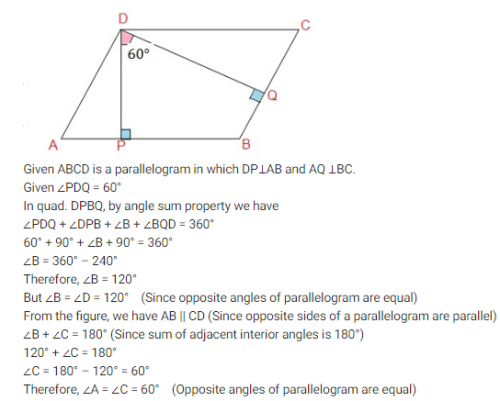QUESTION: 5

If an angle of a parallelogram is two-third of its adjacent angle, then find the smallest angle of the parallelogram.

Solution: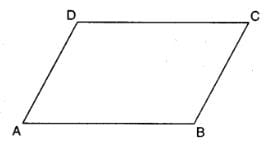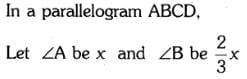⇒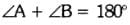⇒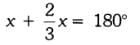⇒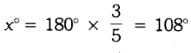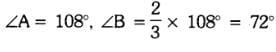QUESTION: 6

If ABCD is a Parallelogram with 2 Adjacent angles ∠A =∠B, then the parallelogram is a

Solution:
QUESTION: 7

Given a triangular prism, then what can we conclude about the lateral faces?

Solution:
In a triangular prism, the bases are triangles and the lateral faces are parallelograms.
Thus it can be both rectangles and parallelograms.
So, option (D) is correct.
If the faces are rectangles, then we call it as right triangular prism
Observe the following figure.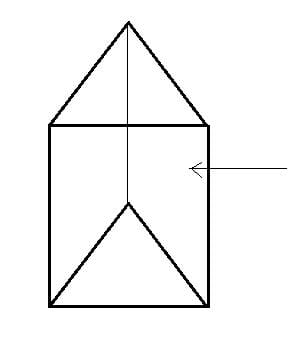It is clear that the faces of the above figure are rectangles and hence it is right triangular prism.
QUESTION: 8

If angles A, B, C and D of the quadrilateral ABCD, taken in order, are in the ratio 3:7:6:4, then ABCD is

Solution:

Given, ratio of angles of quadrilateral ABCD is 3 : 7 : 6 : 4.
Let angles of quadrilateral ABCD be 3x, 7x, 6x and 4x, respectively. We know that, sum of all angles of a quadrilateral is 360°.
3x + 7x + 6x + 4x = 360°
=> 20x = 360°
=> x=360°/20° = 18°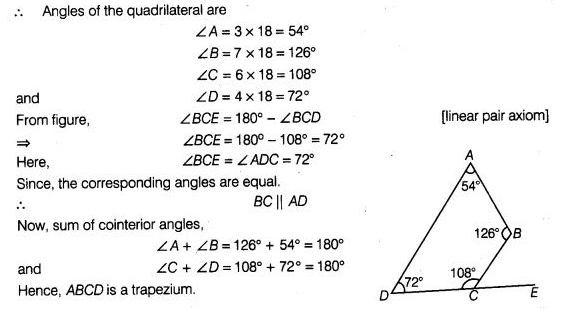QUESTION: 9

ABCD is a Trapezium in which AB∥DC and ∠A = ∠B = 45. Find angles C and D of the Trapezium

Solution:
QUESTION: 10

Angles of a quadrilateral are in the ratio 3 : 6 : 8: 13. The largest angle is :

Solution:

3p + 6p + 8p + 13p = 30p = 360° ⇒ p

= 12° Largest angle is 13p = 13 × 12°

= 156°

QUESTION: 11

If an angle of a parallelogram is two-third of its adjacent angle, the smallest angle of the parallelogram is:

Solution:
QUESTION: 12

Consecutive angles of a Parallelogram are

Solution:
QUESTION: 13

The angles of a quadrilateral are in the ratio 4: 5: 10: 11. The angles are

Solution:

Let x be the common angle among all the four angles of a quadrilateral.

As per angle sum property, we know:

4x+5x+10x+11x = 360°

30x = 360°

x = 12°

Hence, angles are

4x = 4 (12) = 48°

5x = 5 (12) = 60°

10x = 10 (12) = 120°

11x = 11 (12) = 132°

QUESTION: 14

One Angle of a quadrilateral is of 108 and the remaining three angles are equal. Find each of the three equal angles.

Solution: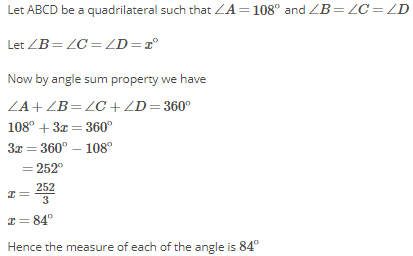QUESTION: 15

ABCD is a Rectangle. Find the values of x and y?

AB =30 DA= 14 DC= x+y CB=x-y

Solution:

ABCD Is A Rectangle, In Which Pair Of Opposite Sides are Equal
So, AB = CD
30 = x + y
14 = x - y
By Solving The Equations
x = 22
y = 8

QUESTION: 16

If area of a Parallelogram with sides ‘a’ and ‘b’ is A and that of a rectangle with sides ‘a’ and ‘b’ is B, then

Solution:
QUESTION: 17

A diagonal of a Rectangle is inclines to one side of the rectangle at an angle of 25. The Acute Angle between the diagonals is :

Solution:
QUESTION: 18

Diagonals of a Parallelogram ABCD intersect at O. If ∠BOC = 90, ∠BDC = 50 then ∠OAB is

Solution:
QUESTION: 19

Angles of a quadrilateral are in the ratio 3 : 4 : 4 : 7. Find all the angles of the quadrilateral.

Solution:
QUESTION: 20

In Triangle ABC which is right angled at B. Given that AB = 9cm, AC = 15cm and D, E are the mid-points of the sides AB and AC res. Find the length of BC?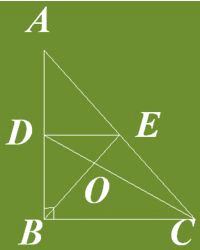Solution:
QUESTION: 21

The Parallel sides of a trapezium are ‘a’ and ‘b’ res. The line joining the mid-points of its non-parallel sides will be

Solution:
QUESTION: 22

If a diagonal AC and BD of a quadrilateral ABCD bisect each other, then ABCD is a

Solution:
QUESTION: 23

Which of the following is not true for the Parallelogram?

Solution:
QUESTION: 24

The angles of the quadrilateral are in the ratios 3 : 5 : 9 : 13. Find all the angles of the Quadrilateral

Solution:
QUESTION: 25

The length of each side of a rhombus is 10cm and one of its diagonal is of length 16cm. The Length of the other Diagonal is:

Solution:

Use pythagoras theorem in right triangle,

102 -[16/2]2 = 100 -64 = 36 = 2 ; hence the other diagonal = 6x2 = 12cmUse Code STAYHOME200 and get INR 200 additional OFF Use Coupon Code